## [NEW PUZZLE] Introducing Notrio, Sudoku's elegant sister :)

For fans of all other kinds of logic puzzles

### [NEW PUZZLE] Introducing Notrio, Sudoku's elegant sister :)

Hi everybody,
Let me introduce Notrio, a new kind of logic puzzle. While belonging to the same category as Sudoku, Notrio has different rules which are simple and elegant, and thus requires completely different techniques to solve. If you want a fresh taste of logic puzzle after Sudoku, and a new challenge for your mind, Notrio is the perfect game for you!

[Notrio rules]
Fill a square grid (size 6x6 to 12x12) with Coral and Turquoise squares such that:
 Three consecutive squares MUST NOT have the same color, and
 Each row and column has an EQUAL number of Coral and Turquoise squares.

[Screenshots]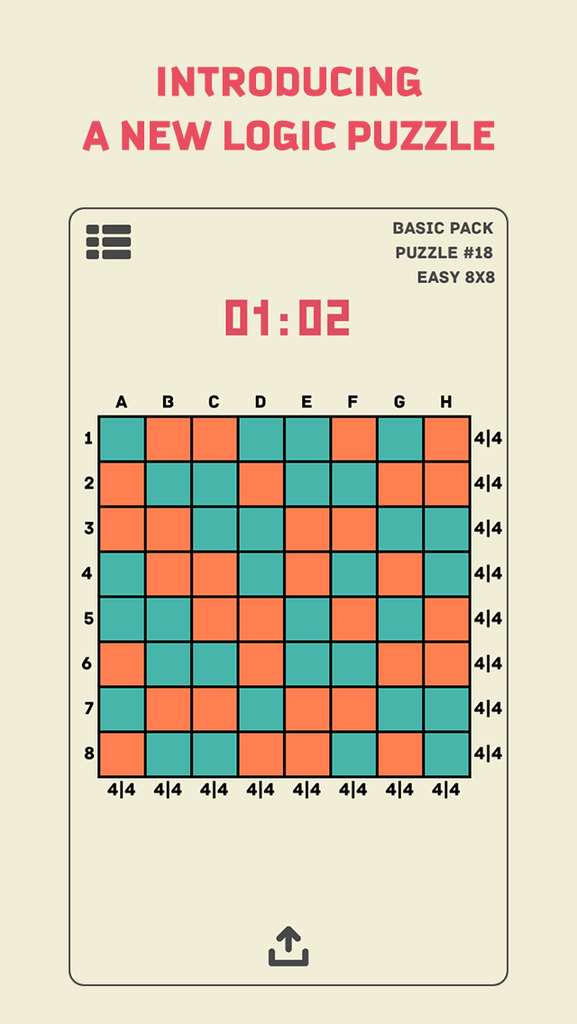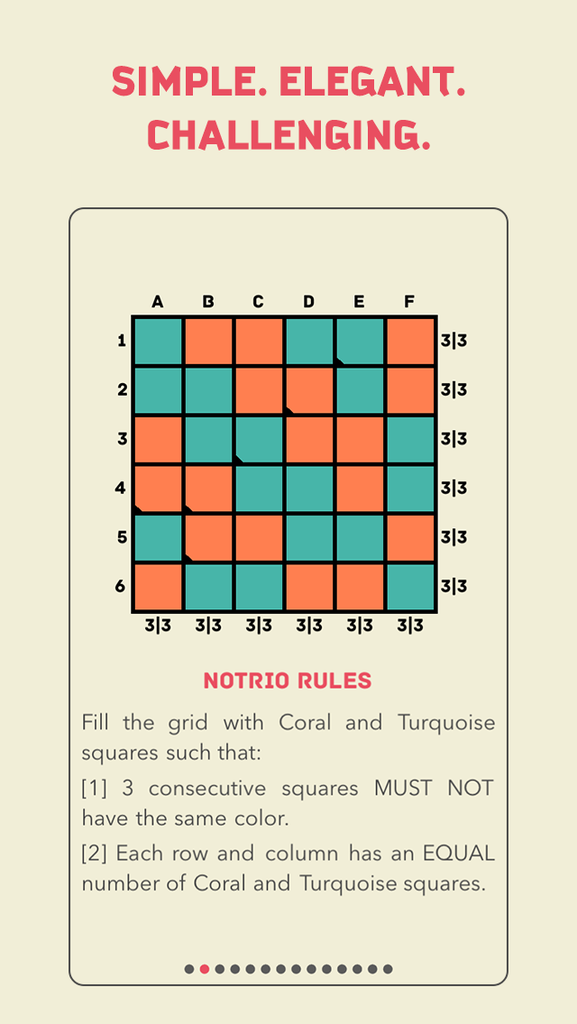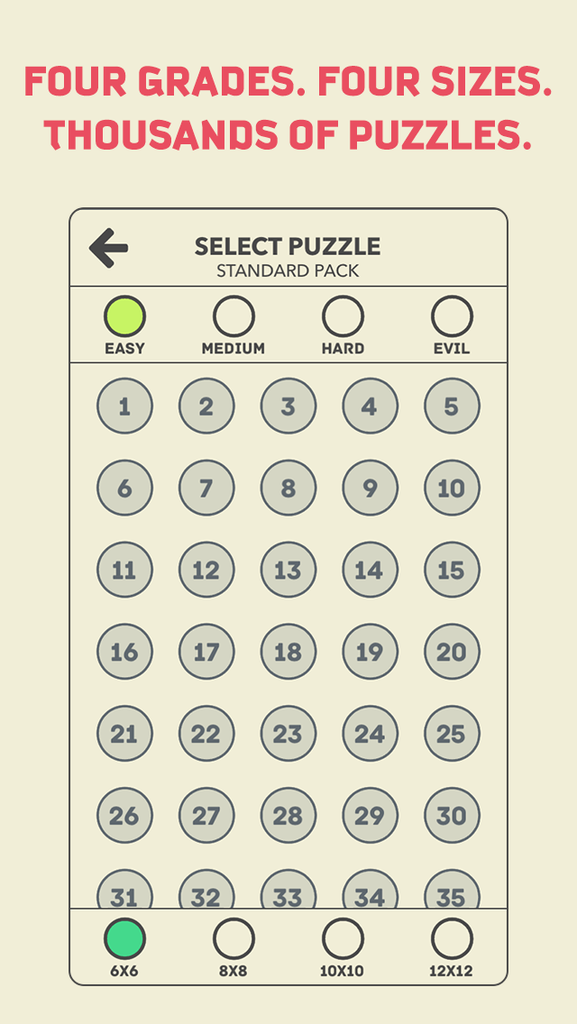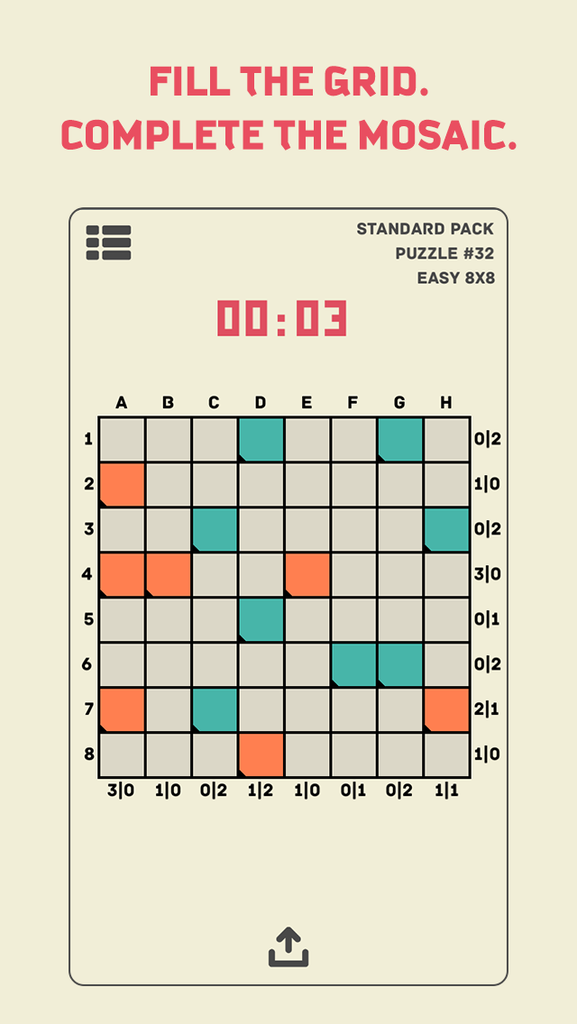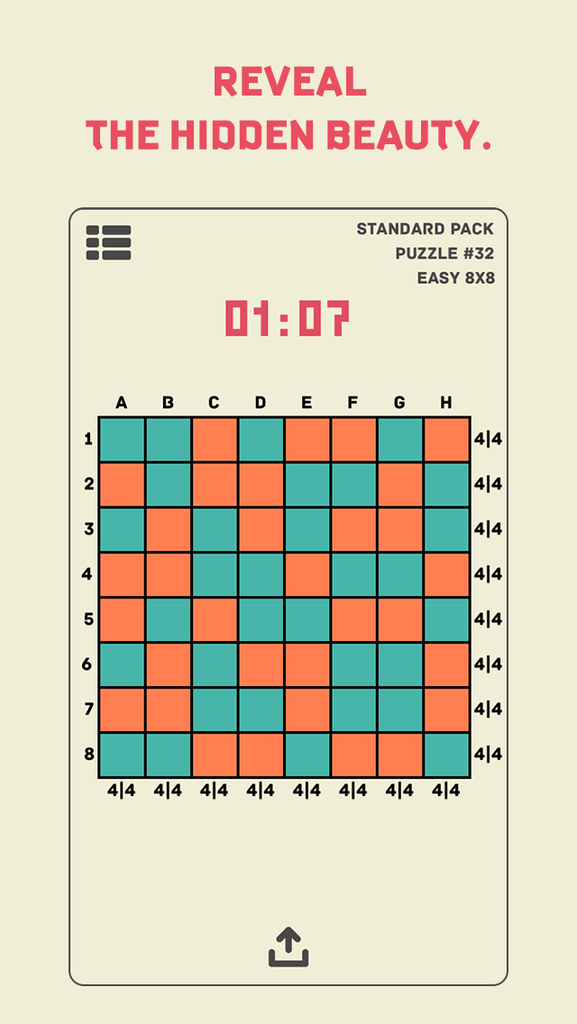iOS: https://itunes.apple.com/us/app/id1007923505

pt5011

Posts: 3
Joined: 20 July 2015

### Re: [NEW PUZZLE] Introducing Notrio, Sudoku's elegant sister

The puzzle looked nice. So I tried to solve manually the above Easy#32.

I consider only two kinds of resolution rules (they can clearly be expressed as pattern-based rules):
1) Basics:
.VV. ==> V'VVV'
V.V ==> VV'V
where
- V stands for any of C (Coral) and T (Turquoise) and V' is the opposite colour,
- in the first rule, one of the "." may be nothing if on edge (in which case the corresponding V' is also nothing).

2) Equal numbers of C and T:
n cells in a row (or column) decided with the same colour V ==> all the rest = V'
(where n = grid_size / 2)

Full resolution path for E#32:

Hidden Text: Show
Code: Select all
`Basics ==>. . . T . . T . C T C . . . . . T C T . . . . TC C T . C . . C . T C T T C . T . . . . C T T C C . T . . . . C. . . C . . . Trow 4 has 4 Ts ==>. . . T . . T . C T C . . . . . T C T . . . . TC C T T C T T C . T C T T C . T . . . . C T T C C . T . . . . C. . . C . . . TBasics ==>. . . T . . T . C T C . . . . . T C T . . . . TC C T T C T T C . T C T T C C T . . . . C T T C C . T . . . . C. . . C . . . Trow 5 has 4 Ts ==>. . . T . . T . C T C . . . . . T C T . . . . TC C T T C T T C C T C T T C C T . . . . C T T C C . T . . . . C. . . C . . . TBasics ==>. . . T . . T . C T C . . . . . T C T C . . . TC C T T C T T C C T C T T C C T T C T C C T T C C C T T C . . C. T C C T . . Trow 7 has 4 Cs ==>. . . T . . T . C T C . . . . . T C T C . . . TC C T T C T T C C T C T T C C T T C T C C T T C C C T T C T T C. T C C T . . TBasics ==>. . . T . . T . C T C . . . . . T C T C . . . TC C T T C T T C C T C T T C C T T C T C C T T C C C T T C T T C. T C C T C C Trow 8 has 4 Cs ==>. . . T . . T . C T C . . . . . T C T C . . . TC C T T C T T C C T C T T C C T T C T C C T T C C C T T C T T CT T C C T C C Tcolumns 1 and 2 have 4 Cs, columns 3 and 4 have 4 Ts ==>T T C T . . T . C T C C . . . . T C T C . . . TC C T T C T T C C T C T T C C T T C T C C T T C C C T T C T T CT T C C T C C TBasics ==>T T C T . . T . C T C C T . . . T C T C . . . TC C T T C T T C C T C T T C C T T C T C C T T C C C T T C T T CT T C C T C C Trow 1 has 4 Ts ==>T T C T C C T CC T C C T . . . T C T C . . . TC C T T C T T C C T C T T C C T T C T C C T T C C C T T C T T CT T C C T C C Tcolumns 5 and 8 have 4 Cs ==>T T C T C C T CC T C C T . . T T C T C T . . TC C T T C T T C C T C T T C C T T C T C C T T C C C T T C T T CT T C C T C C Trow 3 has 4 Ts==>T T C T C C T CC T C C T . . T T C T C T C C TC C T T C T T C C T C T T C C T T C T C C T T C C C T T C T T CT T C C T C C TBasics ==>T T C T C C T CC T C C T T C T T C T C T C C TC C T T C T T C C T C T T C C T T C T C C T T C C C T T C T T CT T C C T C C T`

Of course, the above two types of resolution rules take into account only a small aspect of the original constraints. As a result, there must be lots of puzzles that require more than the above obvious reasoning steps.

Now, I wonder: when the full resolution potential of these rules has been exhausted and the solution is not yet found, what are you left with, except Trial and Error?
denis_berthier
2010 Supporter

Posts: 1987
Joined: 19 June 2007
Location: Paris

### Re: [NEW PUZZLE] Introducing Notrio, Sudoku's elegant sister

. . . . C C
. . . . . .
. T . . C .
. T C . . .
. . C . . T
. . . . . .

We have a partial resolution path, as above:
Hidden Text: Show
Code: Select all
`Basics ==>. . . T C C . C . . T . C T T C C T . T C . . CT C C T C T. . T . . . column 5 has 3 Cs ==>. . . T C C . C . . T . C T T C C T . T C . T CT C C T C T. . T . T . Basics ==>. . . T C C . C . . T . C T T C C T . T C . T CT C C T C T. . T C T . `

But now, what would you do (if not T&E, using e.g. the two possible hypotheses for r4c4) ?
denis_berthier
2010 Supporter

Posts: 1987
Joined: 19 June 2007
Location: Paris

### Re: [NEW PUZZLE] Introducing Notrio, Sudoku's elegant sister

Hi, Denis!
denis_berthier wrote:
Code: Select all
`. . . T C C . C . . T . C T T C C T . T C . T CT C C T C T. . T C T . `

But now, what would you do (if not T&E, using e.g. the two possible hypotheses for r4c4) ?

I see some kind of "chain".
Suppose r2c6 contains "T". Then r2c4 must contain "C", r4c4 must contain "T", r4c1 must contain "C", r2c1 must contain "T". So, we got 3 "T" in r2 row, hence r2c3 must contain "C", but it leads to contradiction - 3 "C" placed in 3 neighbour cells: r2c2, r2c3, r2c4. So, cell r2c6 cannot contain "T" - it contains "C". The rest cells solved by "basic" rules.

Serg
Serg
2018 Supporter

Posts: 723
Joined: 01 June 2010
Location: Russia

### Re: [NEW PUZZLE] Introducing Notrio, Sudoku's elegant sister

Serg wrote:Hi, Denis!
denis_berthier wrote:
Code: Select all
`. . . T C C . C . . T . C T T C C T . T C . T CT C C T C T. . T C T . `

But now, what would you do (if not T&E, using e.g. the two possible hypotheses for r4c4) ?

I see some kind of "chain".
Suppose r2c6 contains "T". Then r2c4 must contain "C", r4c4 must contain "T", r4c1 must contain "C", r2c1 must contain "T". So, we got 3 "T" in r2 row, hence r2c3 must contain "C", but it leads to contradiction - 3 "C" placed in 3 neighbour cells: r2c2, r2c3, r2c4. So, cell r2c6 cannot contain "T" - it contains "C". The rest cells solved by "basic" rules.
Serg

A "chain of inferences", sure, which is exactly the meaning of "T&E based on candidate Tr2c6, using only the Basic and EqualNumber rules".
It's the kind of thing I suggested to do with r4c4 instead of your r2c6.

The example may be too easy. My question was more about the existence of other non "Basic" resolution rules, based on more complex patterns of C/T cells, extending on more than one row or column.
denis_berthier
2010 Supporter

Posts: 1987
Joined: 19 June 2007
Location: Paris

### Re: [NEW PUZZLE] Introducing Notrio, Sudoku's elegant sister

denis_berthier wrote:
Basics ==>
Code: Select all
`. . . T C C . C . . T . C T T C C T . T C . T CT C C T C T. . T C T . `

But now, what would you do (if not T&E, using e.g. the two possible hypotheses for r4c4) ?

Hi Denis and Serg,
Thanks for trying the game. There're several "non basic" techniques which may be helpful when you solve Medium puzzles and above. One of them is LSD (Last Square Deduction, awkward name I know). Anyway, it's a technique in which you inspect any row/column with only one candidate square remained for either color, and find a position for that square which will cause contradiction. Let's take your above example:
- row 1 already contains 2 C's, so based on rule  we know that row 1 can only have one more C (and two more T's).
- now we can fill r1c2 or r1c3 with C and the remained squares with T without causing contradiction (within row 1).
- but if we fill r1c1 with C it will force r1c2 and r1c3 to be T and create 3 T's in a row (r1c2,r1c3,r1c4), which violates rule .
- therefore we can be sure that r1c1 must be a T.
So we're one step further towards the solution, but to solve this particular Hard-grade puzzle you'll need some more "advanced" techniques. Of course, there're many approaches to the solution, for example using the "forcing chains" as Serg did.
I hope you enjoy the logical beauty of this puzzle, and develop more solving techniques on the way!
pt5011

Posts: 3
Joined: 20 July 2015

### Re: [NEW PUZZLE] Introducing Notrio, Sudoku's elegant sister

pt5011 wrote:
denis_berthier wrote:
Basics ==>
Code: Select all
`. . . T C C . C . . T . C T T C C T . T C . T CT C C T C T. . T C T . `

But now, what would you do (if not T&E, using e.g. the two possible hypotheses for r4c4) ?

... a technique in which you inspect any row/column with only one candidate square remained for either color, and find a position for that square which will cause contradiction. Let's take your above example:
- row 1 already contains 2 C's, so based on rule  we know that row 1 can only have one more C (and two more T's).
- but if we fill r1c1 with C it will force r1c2 and r1c3 to be T and create 3 T's in a row (r1c2,r1c3,r1c4), which violates rule .
- therefore we can be sure that r1c1 must be a T.

rule LastV:
. . . V' [already (n/2 - 1) V decided] ==> V' . . V'
I would add it to the Basics, as any rule that can be written within a single row or column.

Do you have more complex examples ?

Note: all the rules I write remain valid under left-right and row-column symmetries
denis_berthier
2010 Supporter

Posts: 1987
Joined: 19 June 2007
Location: Paris

### Re: [NEW PUZZLE] Introducing Notrio, Sudoku's elegant sister

Hi Denis,
Let me introduce some more techniques by solving our example puzzle so far. Here's what we have:
Code: Select all
`T . . T C C . C . . T . C T T C C T . T C . T CT C C T C T. . T C T . `

Now applying the similar technique as above (lastV as you call it), r6c1 cannot be T (will force r2c1, r3c1 & r4c1 to be C), so it must be C.
Code: Select all
`T . . T C C . C . . T . C T T C C T . T C . T CT C C T C TC . T C T . `

Now the interesting part, here's a technique that involves more than 1 row/column:
- we know that r2c1 & r4c1 are different (two last empty squares of column 1)
- similarly r2c4 & r4c4 are different
- similarly r4c1 & r4c4 are different
because of that r2c1 & r2c4 MUST BE DIFFERENT (*)
Next, because r2c2 & r2c5 are different, r2c3 & r2c4 MUST BE DIFFERENT (**) (to comply with rule ).
From (*) and (**), we conclude that r2c1 & r2c3 have the same color, and because r2c2 is already a C, r2c1 & r2c3 must be two T's. That leads to:
Code: Select all
`T . . T C C T C T . T . C T T C C T . T C . T CT C C T C TC . T C T . `

The rest can be solved using basic rules.
This is just one approach to the solution. I hope when the puzzle becomes popular, more people will come up with better techniques, and especially develop advanced techniques to solve Evil-grade puzzles, which I haven't been able to solve without T&E. (If you want to try those Evil ones, they're included in Standard pack, only costs \$1.99).
If you like Notrio please share it with your friends. Thank you very muchpt5011

Posts: 3
Joined: 20 July 2015

### Re: [NEW PUZZLE] Introducing Notrio, Sudoku's elegant sister

pt5011 wrote:Hi Denis,
Let me introduce some more techniques by solving our example puzzle so far. Here's what we have:
Code: Select all
`T . . T C C . C . . T . C T T C C T . T C . T CT C C T C T. . T C T . `

Now applying the similar technique as above (lastV as you call it), r6c1 cannot be T (will force r2c1, r3c1 & r4c1 to be C), so it must be C.
Code: Select all
`T . . T C C . C . . T . C T T C C T . T C . T CT C C T C TC . T C T . `

Thanks for the new example.
So, there are actually (at least) two rules LastV (corresponding to different patterns):
LastV1:   . . . V' [already (n/2 - 1) V decided] ==> V' . . V'
LastV2:   .V.V'. [already (n/2 - 1) V decided] ==> V'V.V'.

pt5011 wrote:Now the interesting part, here's a technique that involves more than 1 row/column:
- we know that r2c1 & r4c1 are different (two last empty squares of column 1)
- similarly r2c4 & r4c4 are different
- similarly r4c1 & r4c4 are different
because of that r2c1 & r2c4 MUST BE DIFFERENT (*)

Using + and - signs for the two (unknown) colours:
r2c4 = -r4c4 = r4c1 = - r2c1

pt5011 wrote:Next, because r2c2 & r2c5 are different, r2c3 & r2c4 MUST BE DIFFERENT (**) (to comply with rule ).
From (*) and (**), we conclude that r2c1 & r2c3 have the same color, and because r2c2 is already a C, r2c1 & r2c3 must be two T's. That leads to:
Code: Select all
`T . . T C C T C T . T . C T T C C T . T C . T CT C C T C TC . T C T . `
.

OK. One has to follow chains of oppositions (which can't easily be written in the same form as the previous rules).
denis_berthier
2010 Supporter

Posts: 1987
Joined: 19 June 2007
Location: Paris